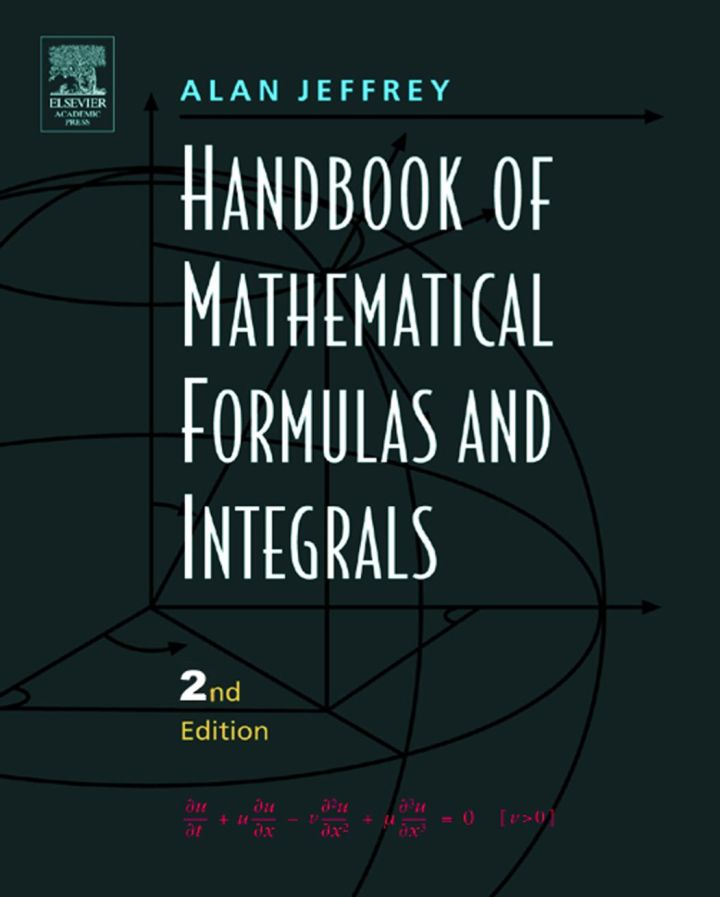# Handbook of Mathematical Formulas and Integrals

## Handbook of Mathematical Formulas and Integrals

By Jeffrey, Alan

### Rent or Buy eTextbook

Expires on Apr 22nd, 2022
\$53.95

Publisher List Price: \$54.99
Savings: \$1.04

The updated Handbook is an essential reference for researchers and students in applied mathematics, engineering, and physics. It provides quick access to important formulas, relations, and methods from algebra, trigonometric and exponential functions, combinatorics, probability, matrix theory, calculus and vector calculus, ordinary and partial differential equations, Fourier series, orthogonal polynomials, and Laplace transforms. Many of the entries are based upon the updated sixth edition of Gradshteyn and Ryzhik's Table of Integrals, Series, and Products and other important reference works.

The Third Edition has new chapters covering solutions of elliptic, parabolic and hyperbolic equations and qualitative properties of the heat and Laplace equation.

Key Features:
* Comprehensive coverage of frequently used integrals, functions and fundamental mathematical results
* Contents selected and organized to suit the needs of students, scientists, and engineers
* Contains tables of Laplace and Fourier transform pairs
* New section on numerical approximation
* New section on the z-transform
* Easy reference system

Subject: Mathematics & Statistics -> Mathematics -> Mathematics General3rd edition
Publisher: Elsevier S & T 12/2003
Imprint: Academic Press
Language: English
Length: 451 pages

ISBN 10: 0123822564
ISBN 13: 9780123822567
Print ISBN: 9780123822567

Live Chats